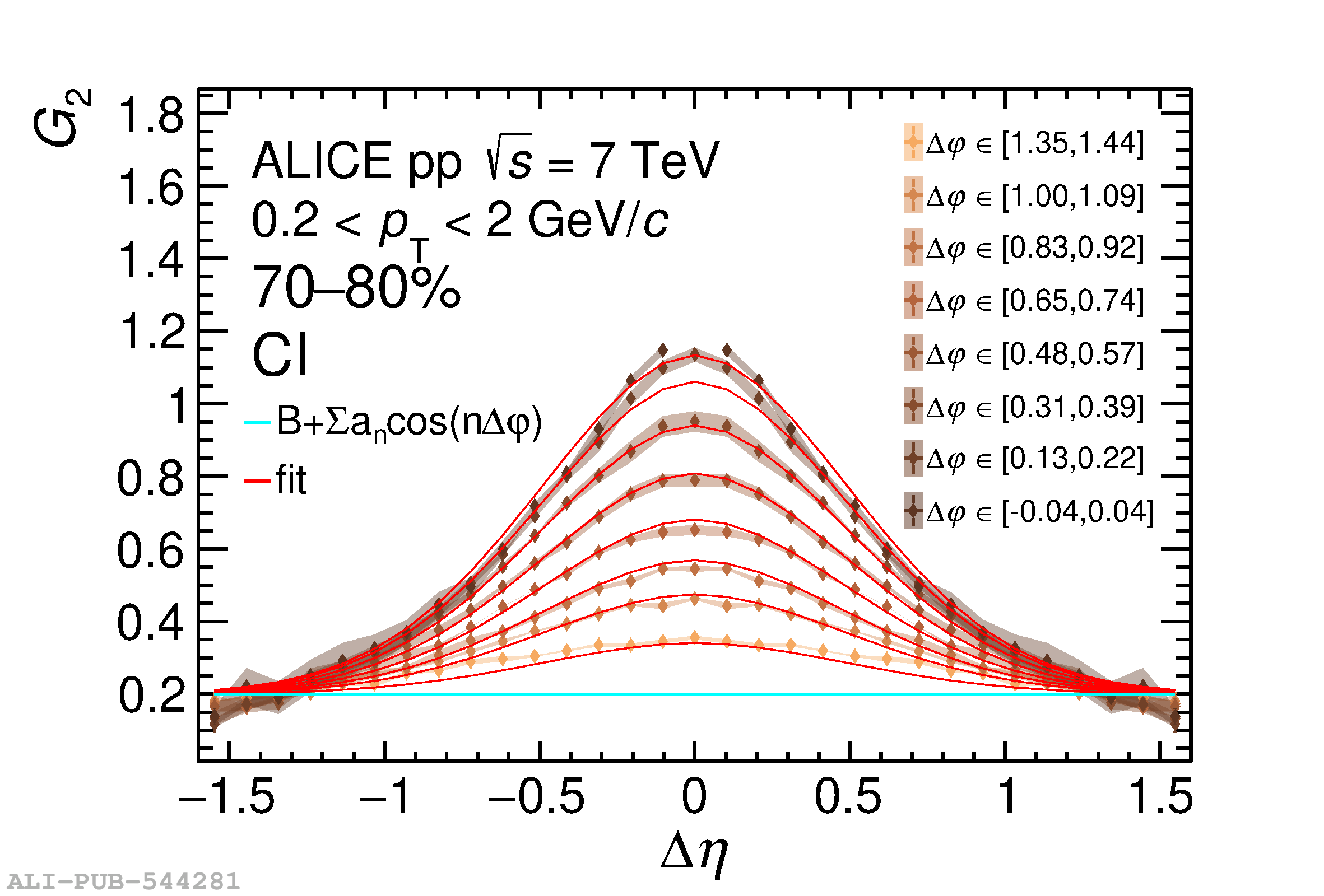# Figure 7

 Longitudinal projections of slices of one azimuthal bin (left) and azimuthal projections of slices of one longitudinal bin (right), for selected bins of the two-particle transverse momentum correlation $G_{2}^{\rm CI}$ and its bidimensional fit using Eq. (\ref{eq:fitfunc}) for the 0--5\% (top) and 70--80\% (bottom) charged particle multiplicity classes in pp collisions at $\sqrt{s}=7\;\text{TeV}$. Vertical bars (mostly smaller than the marker size) and shaded bands represent statistical and uncorrelated systematic uncertainties, respectively.